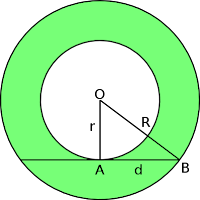## Question

Just because a manifold is topologically non-trivial doesn't necessarily mean it can't be covered by a single chart. In contrast to the circle S1, show that the infinite cylinder R × S1 can be covered by just one chart, by explicitly constructing the map.

The first thing about the chart is that it must be open. That is its boundary is not included. The reason this is important is because the chart must be differentiable everywhere and if any boundary is included, it will not be differentiable there. It would be a sharp edge. This is why S1 cannot be mapped on one chart.Fig 1

Any point on the infinite cylinder can be given coordinates t, θ. t is the distance above or below some arbitrary point, with values from −∞ to +∞ . θ is the angle round the cylinder. 0 ≤ θ < 2π. We cannot simply unroll the cylinder because then we would get an infinitely long strip closed on the left an open on the right as shown in figure 1.

So I started by trying to map to a line which spiralled up the cylinder at some very small angle. Any point on the cylinder would map onto the line and vice-versa. This didn't seem to work.

### Physics forums solution

The first was on physics forums from July 2016 atAnnulus. Wikipedia

Map each line on the cylinder that runs parallel to the cylinder's axis of rotation, to a radial line in the annulus. Then set units along that radial line by parameterizing it in such a way that one approaches but never reaches the outer (inner) circumference of the annulus as t  (t−∞). The parameter t can represent signed distance along the line on the cylinder from an arbitrarily chosen starting point.

This solution might seem to suffer from the problem with the circle S1: Two charts are needed to map it. However this may not the case as I showed in "Commentary 2.2 Covering by a single chart".

Following andrewkirk's instructions we could use the tangent function to parameterise the line as shown belowFig 3. Section through infinite cylinder, showing tangent curve (blue),  and mappings of three points on the cylinder (green)
The tangent curve will rotate through 2π to form a very tall wine glass, without a base and a hole at the bottom. Our original cylinder has radius r and an outer cylinder has a radius R. It limits the outward extent of the curve. The equation of the curve is
t=tan((x- (R+r)/2) π / (R-r))    (1)
It is not trivial to derive the equation. Click Read more below, if you care.

Consider the point P at (t , θ) which maps to P' at (x , θ). From (1)
x = (R+r)/2 +(R-r) (tan-1t) / π   (2)

Therefore, more conventionally, the mapping is
θ' = θ
t' = (R+r)/2 +(R-r) (tan-1t) / π
It is easy to show that this is C. (see pdf for details.)

The most interesting thing about this is that it shows that one can map an infinite area (the cylinder) onto a finite open area - the annulus. It is quite easy to imagine deforming the cylinder into the annulus. The 'mapping' from infinity to the open boundary is also interesting and adds to our intuitive understanding of why an open boundary is differentiable and a closed boundary (or sharp edge) is not.

### University of California Santa Barbara solution

The second solution was at University of California Santa Barbara saved here.
It also contains and answer to Exercise 1.04 on super luminal velocity and I discovered a small treasure trove of answers which I have catalogued in Answers to Exercises..

The answer for the infinite cylinder starts with a half cylinder and ends with a punctured plane. It is more complicated. It should be noted that a punctured plane has striking similarities to an open annulus.

For my pdf on this subject with prettier equations and further links look here
Ex 2.01 One chart for infinite cylinder.pdf

### Foot note 1, the equation of the tangent curve

The tangent curve in Fig 2 must be confined between x = r and x = R in fig 2. But to get the right answer, the operand of the tangent function must lie between -π/2 and π/2. So we must map r to -π/2 and R π/2 to and linearly in between. We use the definition of a straight line y = mx + c and calculate m and c as shown below.